>  Test: BITSAT Mock Test- 8

# Test: BITSAT Mock Test- 8

Test Description

## 150 Questions MCQ Test BITSAT Mock Tests Series & Past Year Papers | Test: BITSAT Mock Test- 8

 1 Crore+ students have signed up on EduRev. Have you?
Test: BITSAT Mock Test- 8 - Question 1

### In a transformer, the output current and voltage are respectively 4 A and 20 V. If ratio of no. of turns in primary to secondary is 2:1, what is the input current and voltage?

Test: BITSAT Mock Test- 8 - Question 2

### What percentage of original radioactive atoms is left after five half lives ?

Test: BITSAT Mock Test- 8 - Question 3

### The hydrogen atom can give spectral lines in the series, Lyman, Balmer and Paschen. Which of the following statements is correct

Test: BITSAT Mock Test- 8 - Question 4
A steady current ι is flowing through a conductor of uniform cross-section. Any segment of the conductor has
Test: BITSAT Mock Test- 8 - Question 5
10 wires (same length, same area, same material)are connected in parallel and each has 1Ω resistance, then the equivalent resistance will be
Test: BITSAT Mock Test- 8 - Question 6
What is the de Broglie wavelength of the α-particle accelerated through a potential difference V
Test: BITSAT Mock Test- 8 - Question 7
The image of the sun is formed on the photosensitive metal with a convex lens and the photoelectric current is I . If the lens is replaced by another similar lens of half the diameter and double the focal length, then photoelectric current will be
Test: BITSAT Mock Test- 8 - Question 8
In an ac circuit, with voltage V and current I the power dissipated is
Test: BITSAT Mock Test- 8 - Question 9

Two thin wire rings each having a radius R are placed at a distance d apart with their axes coinciding. The charges on the two rings are +q and -q. The potential difference between the centres of the two rings is

Test: BITSAT Mock Test- 8 - Question 10
A straight wire of length l and electric dipole moment p is bent to from a semicircle. The new electric dipole moment would be
Test: BITSAT Mock Test- 8 - Question 11

A block of weight 200N is pulled along a rough inclined plane of inclination 45o with the horizontal. If the coefficient of friction is 0.5, the retardation is

Test: BITSAT Mock Test- 8 - Question 12
A particle falls towards earth from infinity. It's velocity on reaching the earth would be
Test: BITSAT Mock Test- 8 - Question 13
On a cold morning, a metal surface will feel colder to touch than a wooden surface because
Test: BITSAT Mock Test- 8 - Question 14

A gas at 27oC has a volume V and pressure P. On heating its pressure is doubled and volume becomes three times. The resulting temperature of the gas will be

Test: BITSAT Mock Test- 8 - Question 15
In a cycltron the angular frequency of the charged particle is independent of the
Test: BITSAT Mock Test- 8 - Question 16
S.I unit of magnetic flux is
Test: BITSAT Mock Test- 8 - Question 17

The surface tension of soap solution is 25*10⁻3 N-m⁻1. The excess pressure inside a soap bubble of diameter 1 cm is

Test: BITSAT Mock Test- 8 - Question 18
The rate of flow of water in a capillary tube of length 'l' and radius 'r' is 'V'. The rate of flow in another capillary tube of length '2l' and radius '2r' for same pressure difference would be
Test: BITSAT Mock Test- 8 - Question 19

A copper wire of length 4 m and area of cross-section 1.2 cm2 is stretched with a force of 4.8 * 103 N. If the Young's modulus for copper is 1.2 * 1011 N-m⁻2, then increase in the length of the wire will be

Test: BITSAT Mock Test- 8 - Question 20

A stone is dropped from rest from the top of a tower 19.6 m high. The distance travelled during the last second of its fall is (given g = 9.8 m/s2)

Test: BITSAT Mock Test- 8 - Question 21
An aeroplane is flying at a constant horizontal velocity of 600 km/hr at an elevation of 6 km towards a point directly above the target on the earth's surface. At an appropeiate time, the pilot releases a ball so that it strikes the target at the earth. The ball will appear to be falling
Test: BITSAT Mock Test- 8 - Question 22

A weightless thread can bear tension upto 37 N. A stone of mass 500 g is tied to it and revolved in a circular path of radius 4 m in a vertical plane. If g = 10 m−s⁻2, then the maximum angular velocity of the stone will be

Test: BITSAT Mock Test- 8 - Question 23
The ratio of the weight of a man in a stationary lift and when it is moving downward with uniform acceleration 'a' is 3:2. The value of 'a' is (g-Acceleration due to gravity of the earth)
Test: BITSAT Mock Test- 8 - Question 24
The hypermetropia is a
Test: BITSAT Mock Test- 8 - Question 25
On a planet, a body takes 2 s to reach the surface when dropped from a height of 8 m. The time period of a simple pendulum of length 1 m on that planet is
Test: BITSAT Mock Test- 8 - Question 26
Which of the following is correct concerning rainbow ?
Test: BITSAT Mock Test- 8 - Question 27
A simple pendulum of length l has a bob of mass m, with a charge q on it. A vertical sheet of charge, with surface charge density σ passes through the point of suspension. At equilibrium, the spring makes an angle θ with the vertical. its time period oscillations is T in this poisiton. Then
Test: BITSAT Mock Test- 8 - Question 28
A body rolls down an inclined plane. If its K.E. of rotational motion is 40% of its K.E. of translation, then the body is
Test: BITSAT Mock Test- 8 - Question 29
If I, α and τ are the moment of inertia, angular acceleration and torque respectively of a body rotating about any axis with angular velocity ω, then
Test: BITSAT Mock Test- 8 - Question 30

A fan is making 600 rev-min⁻1. If it makes 1200 rev-m⁻1, then increase in its angular velocity is

Test: BITSAT Mock Test- 8 - Question 31
The charging of a battery is based on which of the following effect of electric current?
Test: BITSAT Mock Test- 8 - Question 32
The amount of work done in an adiabatic expansion from temperature T to T₁ is
Test: BITSAT Mock Test- 8 - Question 33

For an adiabatic change in a gas, if P, V, T denotes pressure, volume and absolute temperature of a gas at any time and γ is the ratio of specific heats of gas, which of the following is true?

Test: BITSAT Mock Test- 8 - Question 34

The dimensions of the Gravitational constant G are

Test: BITSAT Mock Test- 8 - Question 35
If a star is moving towards the earth, then the spectrum lines are shifted towards
Test: BITSAT Mock Test- 8 - Question 36
In Young's double slit interference experiment the wavelength of light used is 6000$Å$. If the path difference between waves reaching a point P on the screen is 1.5 microns, then at that point P
Test: BITSAT Mock Test- 8 - Question 37
Maximum number of beats frequency heard by a human being is
Test: BITSAT Mock Test- 8 - Question 38
The equation of a progressive wave is y = 8 sin [π(t/10 - x/4)+π/3]. The wavelength of the wave is
Test: BITSAT Mock Test- 8 - Question 39

A body of mass 6 kg is under a force which causes displacement in it given by S = t2/4 mts where t is time. The work done by the force in 2 seconds is

Test: BITSAT Mock Test- 8 - Question 40
A 2 Kg body and a 3 Kg body have equal momentum. If the kinetic energy of 3 Kg body is 10 joules, the kinetic energy of 2 Kg body will be in joules
Test: BITSAT Mock Test- 8 - Question 41
Reaction of phenol with chloroform and sodium hydroxide to give o-hydroxybenzaldehyde involves the formation of
Test: BITSAT Mock Test- 8 - Question 42
Which of the following reagent react differently with HCHO and CH₃CHO and CH₃COCH₃
Test: BITSAT Mock Test- 8 - Question 43
The H-spectrum shows
Test: BITSAT Mock Test- 8 - Question 44
Atoms of different elements having identical mass are known as
Test: BITSAT Mock Test- 8 - Question 45

The order of decreasing stability of the carbanions (CH₃)₃C-(I) ; (CH₃)₂CH-(II) ; CH₃CH₂-(III) ; C₆H₅CH₂-(IV) is

Test: BITSAT Mock Test- 8 - Question 46
The hydrogen phosphate of certain metal has formula MHPO₄. The formula of metal chloride would be
Test: BITSAT Mock Test- 8 - Question 47
Which of the following is an electrophile?
Test: BITSAT Mock Test- 8 - Question 48
A $\sigma$- bond is
Test: BITSAT Mock Test- 8 - Question 49
Chargaff's rule states that in an organism
Test: BITSAT Mock Test- 8 - Question 50
Drying oil invariably contains
Test: BITSAT Mock Test- 8 - Question 51

From the following values of dissociation constants of four acids which value represents the strongest acid ?

Test: BITSAT Mock Test- 8 - Question 52
Which of the following molecules does not have a dipole moment?
Test: BITSAT Mock Test- 8 - Question 53

2 mol of an ideal gas at 27oC expands reversibly from 2L to 20L .The entropy change (R = 2 cal/mol/K) is

Test: BITSAT Mock Test- 8 - Question 54

At certain temperature, 50% of HI is dissociated into H2 and I2, the equilibrium constant is

Detailed Solution for Test: BITSAT Mock Test- 8 - Question 54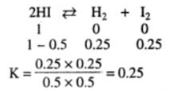Test: BITSAT Mock Test- 8 - Question 55

In gaseous reactions important for the understanding of the upper atmosphere, H2O and O react bimolecularly to form two OH radicals. Δ H for this reaction is 72 kJ at 500 K and Ea is 77 kJ m o l − 1 , then Ea for the bimolecular recombinations of two OH radicals to form H2O and O, is

Test: BITSAT Mock Test- 8 - Question 56
Which of the following elements has the lowest ionisation potential
Detailed Solution for Test: BITSAT Mock Test- 8 - Question 56 The ionisation potential increases from O to F to Ne, because of increasing nuclear charge. The ionisation potential is highest for Ne because of its stable (ns2 np6) electronic configuration. But the ionisation potential of O is lower than N although the nuclear charge of O is higher. This is due to the extra-stability of exactly half-filled 2p-orbitals of N.
Test: BITSAT Mock Test- 8 - Question 57

Given C(s) + O₂(g) → CO₂(g) ; ∆H = −395kJ, S(s) + O₂(g) → SO₂(g);∆H = −295kJ , CS₂(l) + 3O₂(g) → CO₂(g) + 2SO₂(g) ; ∆H = −1110kJ. The heat of formation of CS₂(l) is

Test: BITSAT Mock Test- 8 - Question 58
Amoxillin is semi-synthetic modification of
Test: BITSAT Mock Test- 8 - Question 59
Which type of isomers are [Co(NH₃)₅Br]SO₄ and [Co(NH₃)₅SO₄]Br?
Test: BITSAT Mock Test- 8 - Question 60
The transition elements are more metallic than the representative elements, because they are
Test: BITSAT Mock Test- 8 - Question 61
Chlorine is liberated, when we heat
Test: BITSAT Mock Test- 8 - Question 62
Test: BITSAT Mock Test- 8 - Question 63
The compound that decolourises alkaline KMnO₄ is
Test: BITSAT Mock Test- 8 - Question 64
A gas decolourises alkaline KMnO₄ solution but does not give precipitate with AgNO₃. It is
Test: BITSAT Mock Test- 8 - Question 65
Water softening by Clarke's process uses
Test: BITSAT Mock Test- 8 - Question 66
Precipitation takes place when the ionic product
Test: BITSAT Mock Test- 8 - Question 67
Which of the following molecule having molecular formula C₄H₆O₂ will be optically active?
Test: BITSAT Mock Test- 8 - Question 68
Chloroform on warming with Ag powder gives
Test: BITSAT Mock Test- 8 - Question 69
What are the products formed when ammonia reacts with excess chlorine?
Test: BITSAT Mock Test- 8 - Question 70
Identify the correct statement with respect to carbon monoxide
Test: BITSAT Mock Test- 8 - Question 71

When a vapour, at atmospheric pressure was gradually heated from 25oC its colour was found to deepan at first and then to fade as the temperature was raised above 160oC. At 600oC, the vapour was almost colourless, but its colour deepened when the pressure was raised at this temperature. The vapour was

Test: BITSAT Mock Test- 8 - Question 72
Which of the following is paramagnetic?
Test: BITSAT Mock Test- 8 - Question 73
The chemical used for cooling in refrigeration is
Test: BITSAT Mock Test- 8 - Question 74
Terylene is used for making
Test: BITSAT Mock Test- 8 - Question 75
Which of the following statements is false for alkali metals?
Test: BITSAT Mock Test- 8 - Question 76
The metallic lustre exhibited by sodium is explained by
Test: BITSAT Mock Test- 8 - Question 77

The amount of urea in 100 ml of a solution at 25oC and 20.4 atm. of osmotic pressure is

Test: BITSAT Mock Test- 8 - Question 78

An element (at. mass = 100) having bcc structure has unit cell edge 400 pm. The density of the element is

Test: BITSAT Mock Test- 8 - Question 79
Sulphur forms the chlorides S2Cl2 and SCl2. The equivalent mass of sulphur in SCl2 is 16 g/mole. Therefore the equivalent mass of sulphur in S2Cl2 is
Test: BITSAT Mock Test- 8 - Question 80
The heats of adsorption in physisorption (or physical adsorption) lies in the range (in kJ/mole)
Test: BITSAT Mock Test- 8 - Question 81

Equation to the normal at (3,4) to the circle x2 + y2 = 25 is

Test: BITSAT Mock Test- 8 - Question 82

The area bounded by the curve y = 4x - x2 and x-axis is

Test: BITSAT Mock Test- 8 - Question 83

If n ∈ N then n3+2n is divisible by

Test: BITSAT Mock Test- 8 - Question 84

The circle x2 + y2 - 8x + 4y + 4 = 0 touches

Test: BITSAT Mock Test- 8 - Question 85

If i = √ -1 , the value of i + i2 + i3 - i6 + i8 is

Detailed Solution for Test: BITSAT Mock Test- 8 - Question 85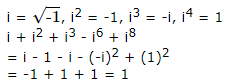Test: BITSAT Mock Test- 8 - Question 86

The solution of the equation (3xy+y2)dx+(x2+xy)dy=0 is

Test: BITSAT Mock Test- 8 - Question 87

The equation of curves which passes from (1,1) and its gradient is ( 2y/x ) is

Detailed Solution for Test: BITSAT Mock Test- 8 - Question 87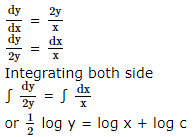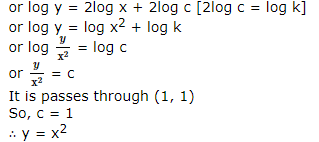Test: BITSAT Mock Test- 8 - Question 88
The solution of differential equation (dy/dx)=[((1+x)y)/((y-1)x)] is
Test: BITSAT Mock Test- 8 - Question 89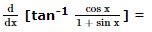Detailed Solution for Test: BITSAT Mock Test- 8 - Question 89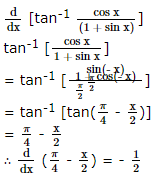Test: BITSAT Mock Test- 8 - Question 90
The function f(x)=x�-3x�-24x+5 is increasing in the interval
Test: BITSAT Mock Test- 8 - Question 91
The area of the region bounded by the curves y = |x-1| and y = 3-|x| is
Test: BITSAT Mock Test- 8 - Question 92
1/(1.2) - 1/(2.3) + 1/(3.4) - 1/(4.5) + ....... is
Test: BITSAT Mock Test- 8 - Question 93

If a flag staff of 6 mt high placed on the top of a tower throws a shadow of 2 √ 3 mt along the ground then the angle that the sun makes with the ground is

Test: BITSAT Mock Test- 8 - Question 94
The equation of the conic with focus at (1, -1), directrix along x - y + 1 = 0 and with eccentricity $\sqrt{2}$ is
Test: BITSAT Mock Test- 8 - Question 95

The area bounded by the curve y2 = 9x and the lines x = 1, x = 4 and y = 0 in the first quadrant is

Test: BITSAT Mock Test- 8 - Question 96

If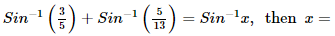Test: BITSAT Mock Test- 8 - Question 97

The function f(x) = cot⁻1 x + x increases in the interval

Test: BITSAT Mock Test- 8 - Question 98

If A and B are two matrices such that AB = B and BA = A, then A2 + B2 is equal to

Test: BITSAT Mock Test- 8 - Question 99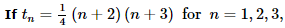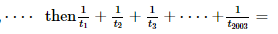Test: BITSAT Mock Test- 8 - Question 100
If the value of a third order determinant is11, then the value of the square of the determinant formed by the co-factors will be
Test: BITSAT Mock Test- 8 - Question 101

The nearest point on the curve y2 = 4x to (2, 1) is

Detailed Solution for Test: BITSAT Mock Test- 8 - Question 101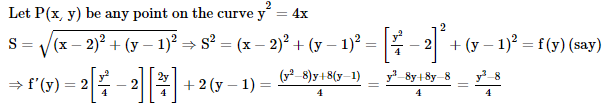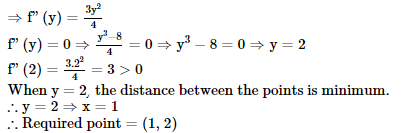Test: BITSAT Mock Test- 8 - Question 102
The solution of the equation ∣ z ∣ = z + 1 + 2 i is
Test: BITSAT Mock Test- 8 - Question 103

The nature of the lines represented by 4x2+12xy+9y2=0 is

Test: BITSAT Mock Test- 8 - Question 104

The line y =mx+1 touches the parabola y2=4x if

Test: BITSAT Mock Test- 8 - Question 105

If lx+my+n=0 is a tangent to the parabola x2=y, then condition of tangency is

Detailed Solution for Test: BITSAT Mock Test- 8 - Question 105

The given Line is lx + my + n = 0 and the parabola x2 = y
Eliminating x from these equation, we get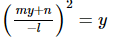or m2y2 + n2 + 2 myn = l2 y
or m2y2 + (2mn - l2)y + n2 = 0
if the line touches the parabola, then the roots of the equation will be equal.
i.e (2mn - l2)2 - 4.m2n2 = 0
or 4.m2n2 + l4 - 4mn.l2 - 4.m2n2 = 0
or l4 - 4mnl2 = 0
or l2 (l2 - 4mn) = 0
or l2 - 4mn = 0
or l2 = 4mn

Test: BITSAT Mock Test- 8 - Question 106
The number of parallelograms that can be formed from a set of four parallel lines intersecting another set of there parallel lines is :
Test: BITSAT Mock Test- 8 - Question 107
A polygon has 44 diagonals. The number of its sides is
Test: BITSAT Mock Test- 8 - Question 108
The probability that at least one of the events A and B occurs is 0.6. If A and B occur simultaneously with probability 0.2, then P(A̅) + P(B̅) is
Test: BITSAT Mock Test- 8 - Question 109
In tossing 10 coins the probabiility of getting exactly 5 heads is
Test: BITSAT Mock Test- 8 - Question 110

In ΔABC , ∑a2 cos2 B − cos2C =

Test: BITSAT Mock Test- 8 - Question 111

If BD, BE and CF are the medians of a ΔABC then
(AD2 + BE2 + CF2) : (BC2 + CA2 + AB2) is equal to

Detailed Solution for Test: BITSAT Mock Test- 8 - Question 111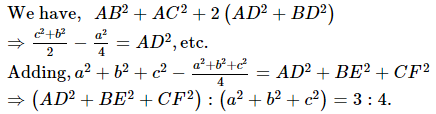Test: BITSAT Mock Test- 8 - Question 112

Let a,b,c be real, if ax2+bx+c = 0 has two real roots α and β, where α<-2 and β>2 then

Test: BITSAT Mock Test- 8 - Question 113
If the sum of the first 2n terms of the A.P. 2, 5, 8,... is equalto the sum of the first n terms of the A.P. 57, 59, 61,.... then n equals
Test: BITSAT Mock Test- 8 - Question 114
If A, B, C be three sets such that A ∪ B = A ∪ C and A ∩ B = A ∩ C, then
Test: BITSAT Mock Test- 8 - Question 115
If log 2,log (2x - 1),log (2x + 3) are in A.P.Then x is equal to
Test: BITSAT Mock Test- 8 - Question 116

If A = {x : x2 - 5x + 6 = 0}, B = {2, 4}, C = {4, 5} then A ∩ (B ∩ C) is

Test: BITSAT Mock Test- 8 - Question 117
The equation of line passing through points (1,2) and (2,5) is
Test: BITSAT Mock Test- 8 - Question 118

The curve x3 - 3xy2 + 2 = 0 and
3x2y - y3 - 2 = 0 cut an angle of

Test: BITSAT Mock Test- 8 - Question 119

The equation of the sphere with centre (3, 6, -4) and touching the plane
2x - 2y - z - 10 = 0 is

Detailed Solution for Test: BITSAT Mock Test- 8 - Question 119

As the required sphere touches the plane
2x - 2y - z - 10 = 0 .....(1)
∴ Radius of the required sphere = ⊥ from the center (3, 6, -4) of the required sphere to the plane (1)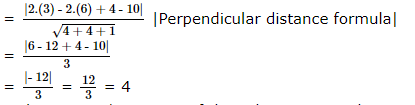∴ The required equation of the sphere is given by
(x - 3)2 + (y - 6)2 + (z + 4)2 = 16 (Central form)
⇒ x2 + 9 - 6x + y2 + 36 - 12y + z2 + 16 + 8z = 16
⇒ x2 + y2 + z2 - 6x - 12y + 8z + 45 = 0

Test: BITSAT Mock Test- 8 - Question 120

If cos α, cos β, cos γ are the d.c. of a line, then value of sinα2+sin2β+sin2γ is

Test: BITSAT Mock Test- 8 - Question 121
If sin2θ=cos3θ and θ is an acute angle, sinθ=
Test: BITSAT Mock Test- 8 - Question 122

Angles A, B and C of a triangle are in A.P. with common difference 15 degree, then angle B is equal to

Test: BITSAT Mock Test- 8 - Question 123

The distance between 6.00 AM and 3.15 P.M. by the tip of the 12 cm long hour hande in a clock is

Detailed Solution for Test: BITSAT Mock Test- 8 - Question 123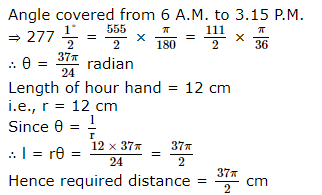Test: BITSAT Mock Test- 8 - Question 124
If P is the resultant of two forces and it is equal to one of them and perpendicular to second, then second force is
Test: BITSAT Mock Test- 8 - Question 125
If three vectors a=2i-j+k, b=i+2j-3k, c=3i+λj+5k are coplanar, then λ=
Test: BITSAT Mock Test- 8 - Question 126
Find the Antonym of the word occurring in the sentence in capital letter as per the context.
He is a GENEROUS man.
Detailed Solution for Test: BITSAT Mock Test- 8 - Question 126 'Generous' means willing to give freely and 'Stingy' means not giving willingly
Test: BITSAT Mock Test- 8 - Question 127
Find the antonym of NETTLE.
Test: BITSAT Mock Test- 8 - Question 128
Fill in the blank with appropriate word.
My father was too.......to push open the heavy door.
Test: BITSAT Mock Test- 8 - Question 129
Fill in the blank with appropriate word.
In a little publicised deal, Pepsi Cola has.......the entire soft drink market in Afghanistan.
Test: BITSAT Mock Test- 8 - Question 130
Choose the one which can be substituted for the given words/sentences
Very pleasing to eat.
Test: BITSAT Mock Test- 8 - Question 131
Choose the one which can be substituted for the given words/sentences :
Medical study of the skin and its diseases
Test: BITSAT Mock Test- 8 - Question 132
Spot the error.
Test: BITSAT Mock Test- 8 - Question 133
Spot the error.
Test: BITSAT Mock Test- 8 - Question 134
Spot the error.
Detailed Solution for Test: BITSAT Mock Test- 8 - Question 134 The correct statement is 'he returned'
Test: BITSAT Mock Test- 8 - Question 135
Find the Synonym of the word occurring in the sentence in capital letter as per the context.
His descriptions are VIVID.
Detailed Solution for Test: BITSAT Mock Test- 8 - Question 135 'Vivid' means clear
Test: BITSAT Mock Test- 8 - Question 136
Find the Synonym of the word occurring in the sentence in capital letter as per the context.
Please do not give any more FLIMSY pretexts for not having done the work.
Detailed Solution for Test: BITSAT Mock Test- 8 - Question 136 'Flimsy' means not strong enough; difficult to believe
Test: BITSAT Mock Test- 8 - Question 137
Complete the analogous pair.
Sand : Seed : : Spend : ?
Test: BITSAT Mock Test- 8 - Question 138
Choose the word which is least like the other words in the group .
Test: BITSAT Mock Test- 8 - Question 139

Find the missing character.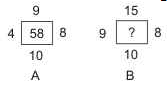Detailed Solution for Test: BITSAT Mock Test- 8 - Question 139

In fig. (A), (9 x 10)   − (4 x 8)  = 58.
In fig. (B), missing number = (15 x 10)   − (9 x 8)   = 150 − 72 = 78.

Test: BITSAT Mock Test- 8 - Question 140

Group the following figures into three classes on the basis of identical properties.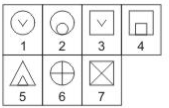Test: BITSAT Mock Test- 8 - Question 141
Find out the term in the number series which is wrong.
3, 10, 27, 4, 16, 64, 5, 25, 125
Test: BITSAT Mock Test- 8 - Question 142
What will be the next term in : BDF, CFI, DHL, ?
Test: BITSAT Mock Test- 8 - Question 143

Some people believe that they are masters of their own fate . Other people see themselves as pawns of fate , believing that what happens to them in their lives is due to luck or chance. The first type, those who believe that they control their destinies, have been labelled internals, whereas the latter, who see their lives as being controlled by outside forces, have been called externals. Internals search more actively for information before making a decision , are more motivated to achieve , and make a greater attempt to control their environment. Externals, however, are more compliant and willing to follow directions. Therefore, internals do well on sophisticated tasks-which include most managerial and professional jobs-that require complex information processing and learning. In addition , internals are more suited to jobs that require initiative and independence of action . Almost all successful sales people, for instance are internals. In contrast, externals should do well on jobs that are well structured and routine and in which success depends heavily on complying with the directions of others.

Q. Which one of the following is not correct ?
Internals: